# C Program for Insertion Sort

In this article, we will learn how to create a program in C that sorts an array in ascending order using the insertion sort technique. In addition, we have created a function that can be used to sort any given array (by the user at run-time) in ascending order using the insertion sort technique.

But before going through the program, if you are not aware of how an insertion sort actually works, then I recommend that you go through the step-by-step workings of an insertion sort. Let's move on and implement it in a C program.

## C Insertion Sort

Let's go through the insertion-sort program first. Later on, I'll explain each and every step involved in this program. The question is, "Write a program in C that sorts any given array in ascending order using the insertion sort technique." The answer to this question is:

```#include<stdio.h>
#include<conio.h>
int main()
{
int arr, size, i, j, k, element, index;
printf("Enter Array Size: ");
scanf("%d", &size);
printf("Enter %d Array Elements: ", size);
for(i=0; i<size; i++)
scanf("%d", &arr[i]);
for(i=1; i<size; i++)
{
element = arr[i];
if(element<arr[i-1])
{
for(j=0; j<=i; j++)
{
if(element<arr[j])
{
index = j;
for(k=i; k>j; k--)
arr[k] = arr[k-1];
break;
}
}
}
else
continue;
arr[index] = element;
}
printf("\nSorted Array:\n");
for(i=0; i<size; i++)
printf("%d ", arr[i]);
getch();
return 0;
}```

Because the above program was written in the Code::Blocks IDE, you will receive the following output after a successful build and run. Here is the first snapshot of the sample run: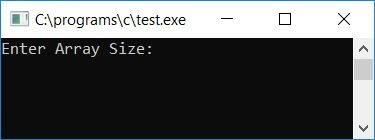Now supply the size of the array, say 5, and enter any 5 array elements. And then press the ENTER key to sort that array. Here is the second snapshot of the sample run: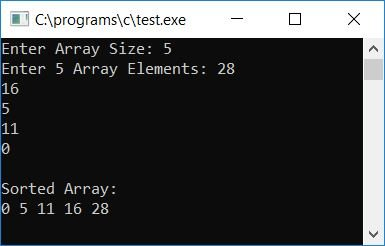Let's take another sample run. Here is the final snapshot of the sample run: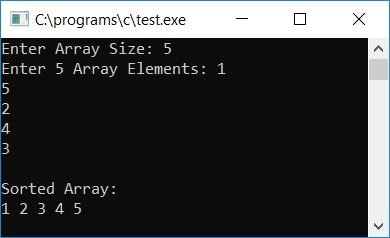#### Program Explained

• Receive the size of an array, and then receive elements of that size.
• That is, if the user supplies 5 as the array size, then ask him/her to enter any 5 elements for that array.
• Create a for loop that runs from 1 to one less than the size of the array.
• Inside the loop, initialize the current element, that is, the element present at ith index of the array, to any variable, say "element."
• Use an if statement to check whether the current element is less than the previous element or not.
• If it is, then program flow goes inside the if block.
• Inside the if block, create another for loop that runs from start (zero) to less than or equal to the value of i (the outer loop variable).
• Inside this loop, check whether the value of the element is less than the value of arr[j] or not.
• If it is, then initialize the current index to a variable, say index, and push all the elements to its next index one by one using a for loop.
• That is, create a for loop that starts from the value of i and runs until it is greater than the value of j (the outer loop variable).
• Never forget to use the break keyword to break out of the for loop.
• And if the statement of if's condition evaluates to false, then use the continue keyword to tell the compiler to go back to the outer for loop. That is, increment its variable and continue to do the same process as told above.
• After performing this and continuing to increment the first for loop variable (i), initialize the value of the element variable at arr[index].
• For example, if the user supplied an array: 1, 5, 2, 4, 3.
• Therefore, at the first run of the loop,
• The loop variable i is initialized to 1, and i<size, or 1<5, evaluates to true. Therefore, program flow goes inside the loop.
• The value at arr[i], arr, or 5 is set to element.
• And element<arr[i-1] or 5<arr or 5<1 evaluates to false, therefore program flow goes to else block, and using continue keyword, program flow goes back to the first for loop.
• That is, there was no any swapping, so the array remains in its original order of 1 5 2 4 3.
• As the program flow again came back to the for loop and incremented the value of i.
• Now that i is equal to 2, i<size or 2<5 evaluates to true. Therefore, program flow goes inside the loop again.
• The value at arr[i] or arr or 2 is set to element.
• And element<arr[i-1] or 2<arr or 2<5 evaluates to true, therefore, program flow goes inside the if block.
• The value of j (the loop variable of the inner for loop) gets initialized with 0, and j<=i or 0<=2 evaluates to true, therefore program flow goes inside the loop.
• And because element<arr[j] or 2<1 evaluates to false, program flow returns to the inner for loop and increments the value of j.
• Now that j is 1, j<=i or 1<=2 evaluates to true. Therefore, program flow goes inside the loop.
• And again, element<arr[j] or 2<5 evaluates to true, therefore program flow goes inside the if block.
• And j or 1 is assigned to the index variable. And the value of i or 2 gets initialized to k (the loop variable).
• And k>j or 2>1 evaluates to true, therefore program flow goes inside the loop, and arr[k-1] or arr or 5 gets initialized at arr[k] or arr.
• The value of k now gets decremented and becomes 1. And k>j or 1>1 returns false.
• Therefore, program flow goes out of this loop, and finds the break keyword. The program flow returns to the first for loop.
• Now the new array is 1 2 5 4 3.
• There, the value of i again gets incremented, and i<size or 3<5 evaluates to true. Therefore, program flow again goes inside the loop and follows the same procedure as told above to sort the array as per the insertion sort technique.

### After each Insertion Sort, print the array

If you want to see the step-by-step array after each sorting on the output screen, then you can modify the above program with the following one:

```#include<stdio.h>
#include<conio.h>
int main()
{
int arr, size, i, j, k, element, index;
printf("Enter Array Size: ");
scanf("%d", &size);
printf("Enter %d Array Elements: ", size);
for(i=0; i<size; i++)
scanf("%d", &arr[i]);
for(i=1; i<size; i++)
{
element = arr[i];
if(element<arr[i-1])
{
for(j=0; j<=i; j++)
{
if(element<arr[j])
{
index = j;
for(k=i; k>j; k--)
arr[k] = arr[k-1];
break;
}
}
}
else
continue;
arr[index] = element;
printf("\nStep %d: ", i);
for(j=0; j<size; j++)
printf("%d ", arr[j]);
printf("\n");
}
printf("\nSorted Array:\n");
for(i=0; i<size; i++)
printf("%d ", arr[i]);
getch();
return 0;
}```

Here is the final snapshot of the sample run:Here is another final snapshot of another sample run: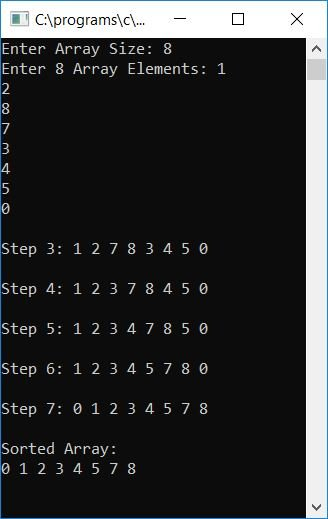### Insertion Sort in C using the while loop

Let's create another program that also sorts any given array in ascending order as per the insertion sort technique. Here we have used the while loop to shorten the code:

```#include<stdio.h>
#include<conio.h>
int main()
{
int arr, i, j, elem;
printf("Enter any 5 array elements: ");
for(i=0; i<5; i++)
scanf("%d", &arr[i]);
for(i=1; i<5; i++)
{
elem = arr[i];
j = i-1;
while((elem<arr[j]) && (j>=0))
{
arr[j+1] = arr[j];
j--;
}
arr[j+1] = elem;
}
printf("\nSorted Array in ascending order:\n");
for(i=0; i<5; i++)
printf("%d ", arr[i]);
getch();
return 0;
}```

Here is the final snapshot of the sample run: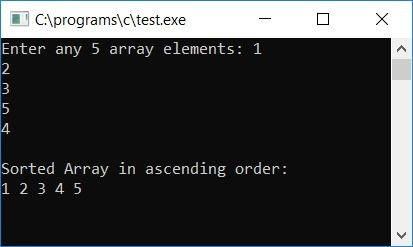### C Insertion Sort with Function

Let's create a function that takes any two arguments (an array and its size) and sorts that array in ascending order as per the insertion sort technique. Here is the program that works the same as above, except that here we have created a function that performs sorting:

```#include<stdio.h>
#include<conio.h>
void insertsort(int arr[], int size);
int main()
{
int arr, size, i;
printf("How many element you want to store? ");
scanf("%d", &size);
printf("Enter any %d array elements: ", size);
for(i=0; i<size; i++)
scanf("%d", &arr[i]);
insertsort(arr, size);
printf("\nSorted Array in ascending order:\n");
for(i=0; i<size; i++)
printf("%d ", arr[i]);
getch();
return 0;
}
void insertsort(int arr[], int size)
{
int i, elem, j;
for(i=1; i<size; i++)
{
elem = arr[i];
j = i-1;
while((elem<arr[j]) && (j>=0))
{
arr[j+1] = arr[j];
j--;
}
arr[j+1] = elem;
}
}```

As you can see from the above program, we have declared a function at the start of the program, that is, before the main() function. This function accepts any two arguments, the first of which is the array and the second of which is its size. We have defined the function so that after calling the function, the program successfully performs the action as defined in the function definition. Here is the final snapshot of the sample run:#### The same program in different languages

C Quiz

« Previous Program Next Program »